Trigonometric Ratios Contents w Introduction to Trigonometric Ratios

• Slides: 28Trigonometric Ratios Contents w Introduction to Trigonometric Ratios w Unit Circle w Adjacent , opposite side and hypotenuse of a right angle triangle. w Three types trigonometric ratios w Conclusion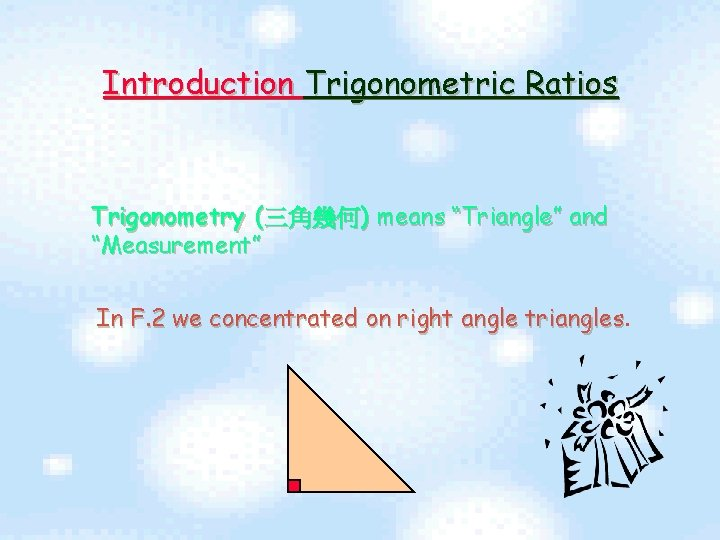Introduction Trigonometric Ratios Trigonometry (三角幾何) means “Triangle” and “Measurement” In F. 2 we concentrated on right angle trianglesUnit Circle A Unit Circle Is a Circle With Radius Equals to 1 Unit. (We Always Choose Origin As Its centre) Y 1 units x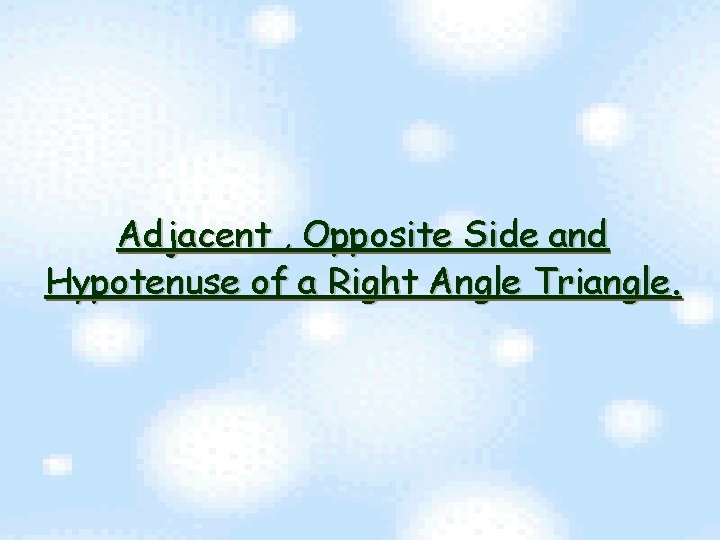Adjacent , Opposite Side and Hypotenuse of a Right Angle Triangle.ot p hy se u en Opposite side Adjacent side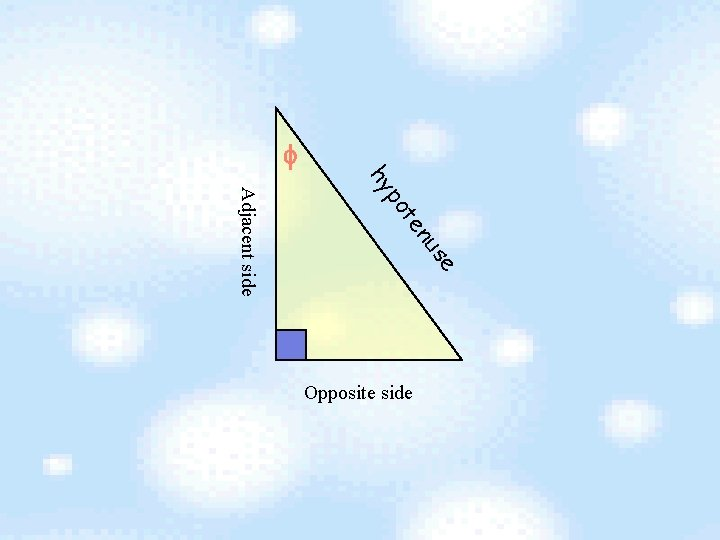ot p hy se u en Adjacent side Opposite side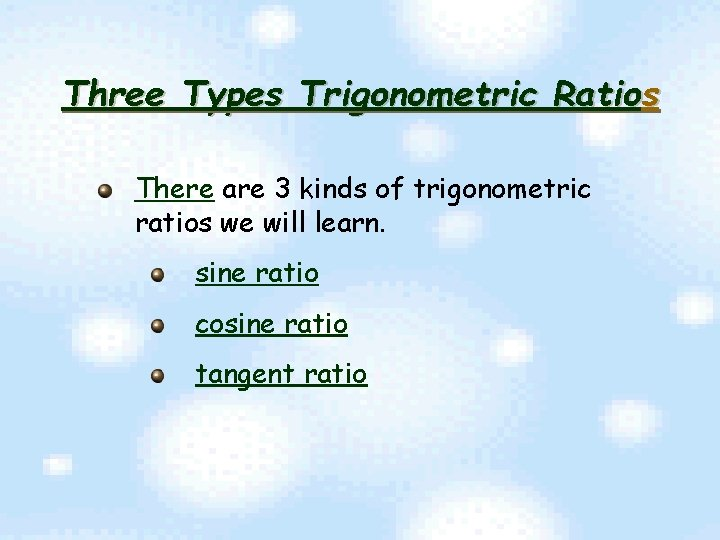Three Types Trigonometric Ratios There are 3 kinds of trigonometric ratios we will learn. sine ratio cosine ratio tangent ratioSine Ratios Ø Definition of Sine Ratio. Ø Application of Sine Ratio.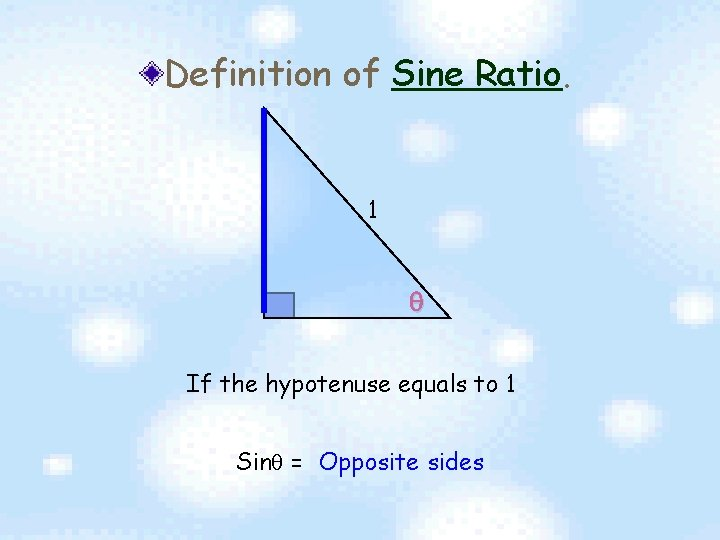Definition of Sine Ratio. 1 If the hypotenuse equals to 1 Sin = Opposite sides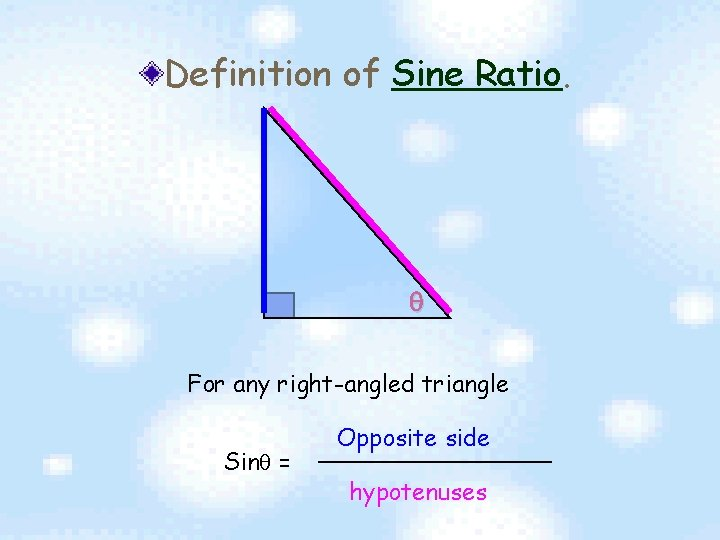Definition of Sine Ratio. For any right-angled triangle Sin = Opposite side hypotenusesExercise 1 In the figure, find sin Sin = = = Opposite Side hypotenuses 4 7 34. 85 (corr to 2 d. p. ) 4 7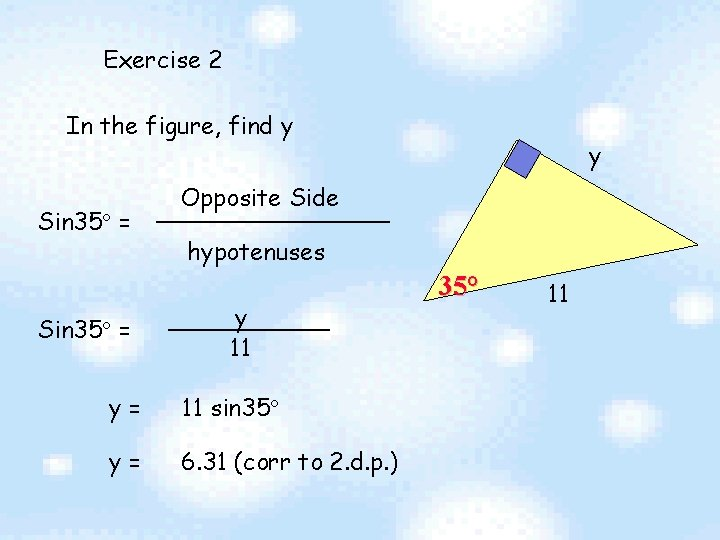Exercise 2 In the figure, find y Sin 35 = y Opposite Side hypotenuses y 11 y= 11 sin 35 y= 6. 31 (corr to 2. d. p. ) 35° 11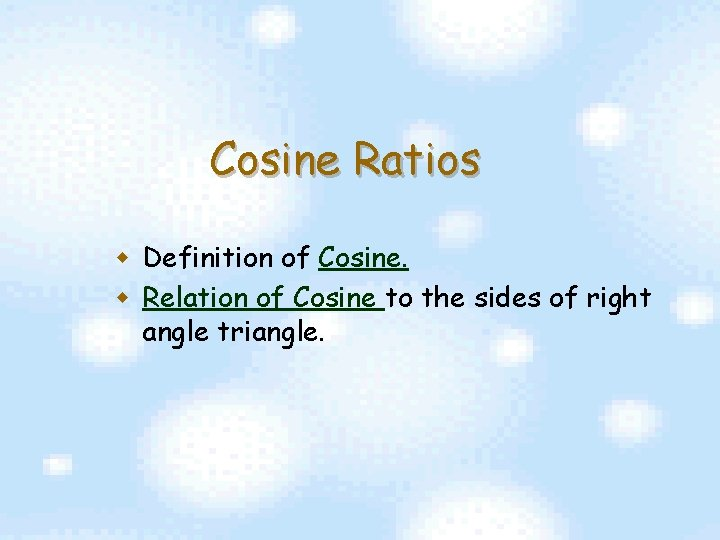Cosine Ratios w Definition of Cosine. w Relation of Cosine to the sides of right angle triangle.Definition of Cosine Ratio. 1 If the hypotenuse equals to 1 Cos = Adjacent Side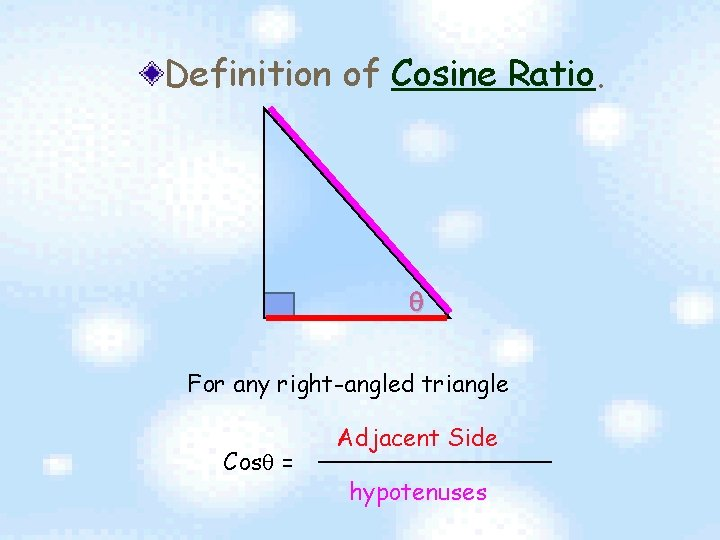Definition of Cosine Ratio. For any right-angled triangle Cos = Adjacent Side hypotenusesExercise 3 In the figure, find cos = = = adjacent Side hypotenuses 3 8 67. 98 (corr to 2 d. p. ) 3 8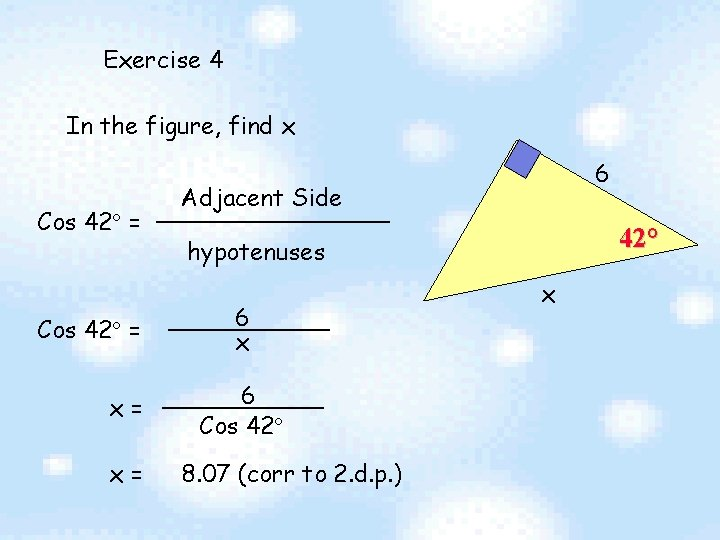Exercise 4 In the figure, find x Cos 42 = x= x= 6 Adjacent Side 42° hypotenuses 6 x 6 Cos 42 8. 07 (corr to 2. d. p. ) x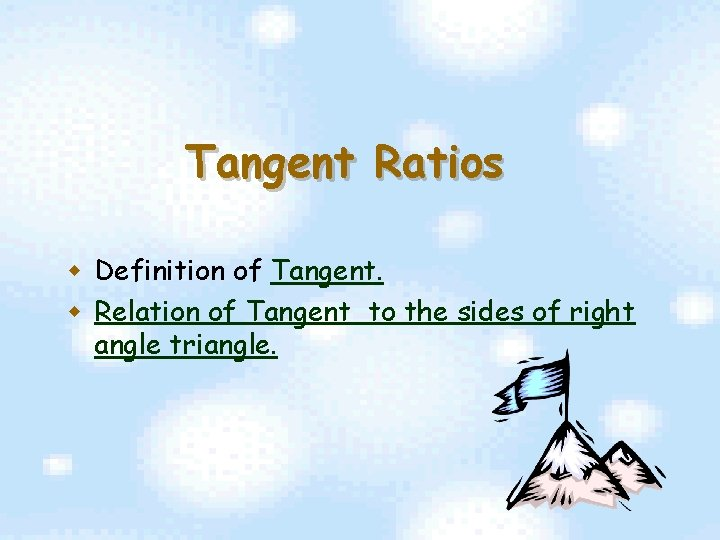Tangent Ratios w Definition of Tangent. w Relation of Tangent to the sides of right angle triangle.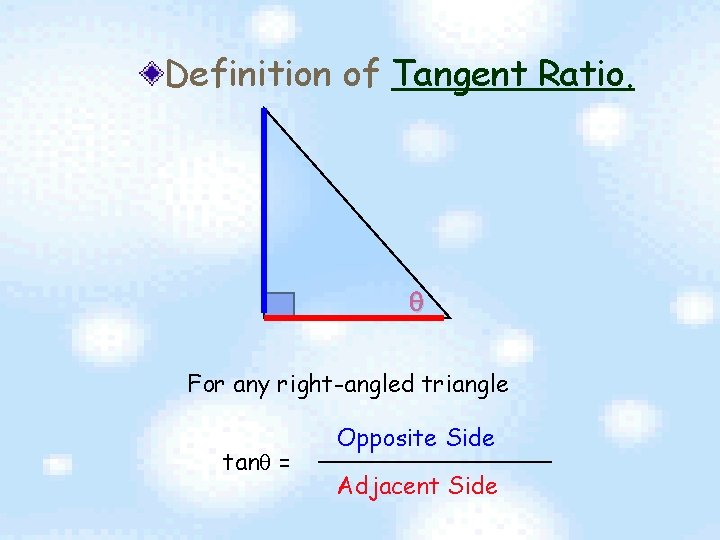Definition of Tangent Ratio. For any right-angled triangle tan = Opposite Side Adjacent Side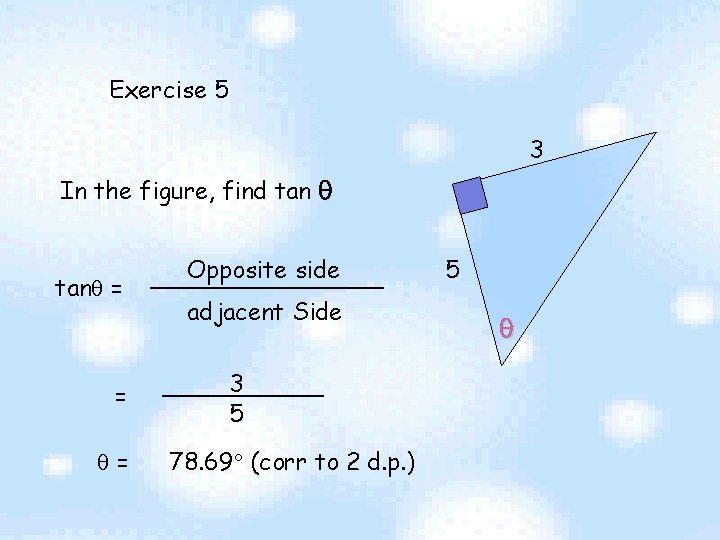Exercise 5 3 In the figure, find tan = = = Opposite side adjacent Side 3 5 78. 69 (corr to 2 d. p. ) 5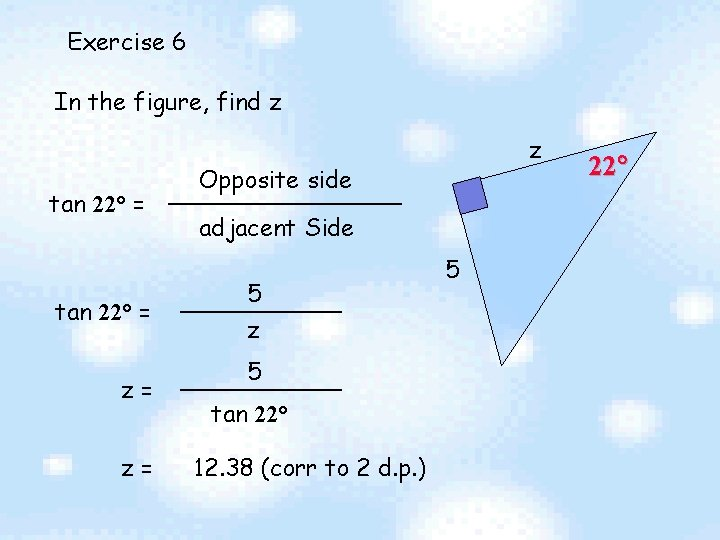Exercise 6 In the figure, find z tan 22 = z= z= z Opposite side adjacent Side 5 z 5 tan 22 12. 38 (corr to 2 d. p. ) 5 22Conclusion Make Sure that the triangle is right-angledEND